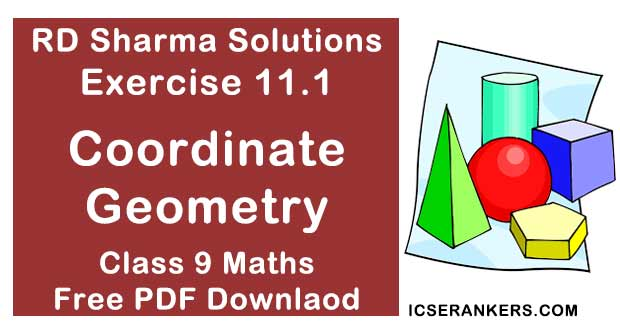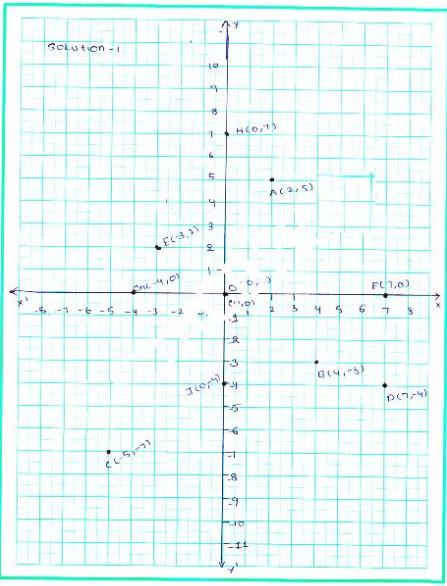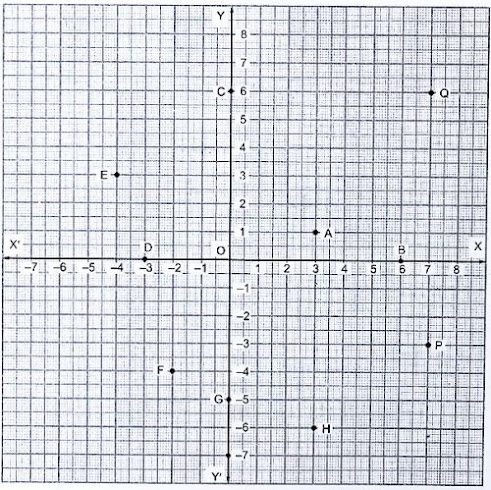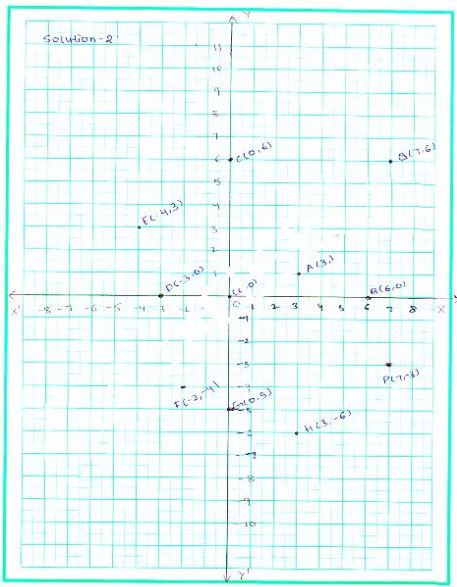# Chapter 11 Coordinate Geometry RD Sharma Solutions Exercise 11.1 Class 9 MathsChapter Name RD Sharma Chapter 11 Coordinate Geometry Exercise 11.16 Book Name RD Sharma Mathematics for Class 10 Other Exercises No Other Exercises Related Study NCERT Solutions for Class 10 Maths

### Exercise 11.1 Solutions

1. Plot the following points on the graph paper :
(i) (2, 5)
(ii) (4, -3)
(iii) (-5, -7)
(iv) (7, -4)
(v) (-3, 2)
(vi) (7, 0)
(vii) (-4, 0)
(viii) (0, 7)
(ix) (0, -4) (x) (0, 0)
Solution
The given points are a(2, 5),  b(4, -3), c(-5, 7),  d(7, -4),  e(-3, 2),  f(7, 0), g(-4, 0), h(0, 7) & i(0, -4)
The given points represented on the graph as follows:2. Write the coordinates of each of the following points marked in the graph paper :Solution
The given points are A (3, 1) B (6, 0), c(0, 6) d(-3, 0) e(-4, 3) f(-2, -4) g(0, -5) h(3, -6) p(7,−3) & q(7, 6)
The Graph of coordinates of given points as follows: